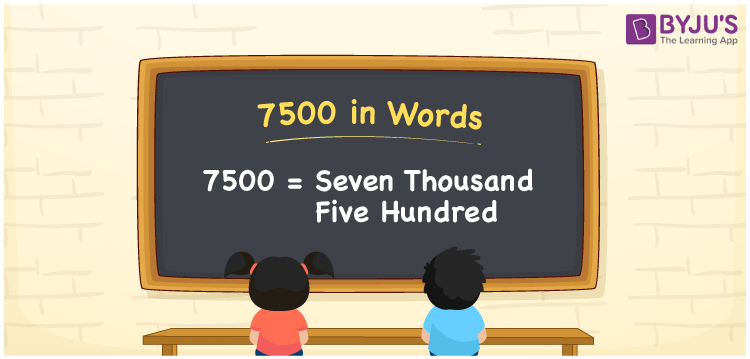The number 7500 in words is written as seven thousand five hundred. The place values such as ones, tens, and hundreds are used to write the number name of 7500. As a result, the place value table proves useful when writing the number 7500 in English. For instance, if you spent Rs. 7500 for a coat, you can write “I spent Rs. Seven thousand five hundred for a coat.” In this topic, we’ll look at how to spell 7500 in English words and also how to express the number 7500 in words in detail.

 7500 in Words: Seven thousand five hundred Seven thousand five hundred in Numerical Form: 7500

## 7500 in English Words## How to Write 7500 in Words?

Identify the place value of each digit in the given number while writing 7500 in Words. Below is the place value chart for the number 7500:

 Thousands Hundreds Tens Ones 7 5 0 0

As a result, the extended form of 7500 is:

= 7 × Thousand + 5 × Hundred + 0 × Ten + 0 × One

= 7 × 1000 + 5 × 100 + 0 × 10 + 0 × 1

= 7500

= Seven thousand five hundred

Therefore, 7500 in words is Seven thousand five hundred.

7500 is the natural number that follows 7499 but precedes 7501.

7500 in words – Seven thousand five hundred

Is 7500 an odd number? – No

Is 7500 an even number? – Yes

Is 7500 a perfect square number? – No

Is 7500 a perfect cube number? – No

Is 7500 a prime number? – No

Is 7500 a composite number? – Yes

## Frequently Asked Questions on 7500 in Words

Q1

### How to write 7500 in words?

7500 in words is seven thousand five hundred.

Q2

### Simplify 8000-500, and express in words.

Simplifying 8000-500, we get 7500. Therefore, 7500 in words is seven thousand five hundred.

Q3

### Convert seven thousand five hundred into numbers.

Seven thousand five hundred in numbers is 7500.